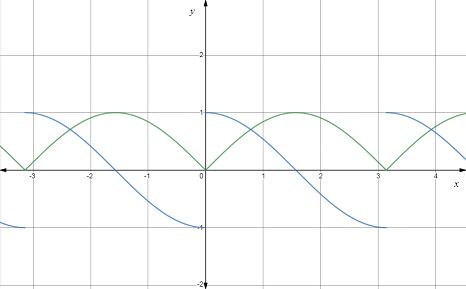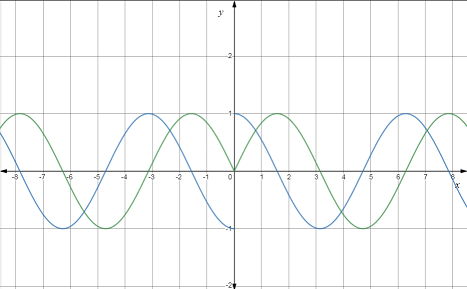# Explain the proof of the given relation using chain rule.### Single Variable Calculus: Concepts...

4th Edition
James Stewart
Publisher: Cengage Learning
ISBN: 9781337687805### Single Variable Calculus: Concepts...

4th Edition
James Stewart
Publisher: Cengage Learning
ISBN: 9781337687805

#### Solutions

Chapter 3.4, Problem 92E

a.

To determine

## Explain the proof of the given relation using chain rule.

Expert Solution

### Explanation of Solution

Given:

The given relationis ddx|x|=x|x| , where |x|=x2 .

Calculation:

ddx|x|=x|x|

From L.H.S

ddx|x|

|x|=x2

ddx|x|=ddxx2

Apply chain rule.

Let f=a,a=x2

ddx|x|=dda(a)ddx(x2)

Use derivative rule.

ddx(x)=12xandddx(xn)=nxn1 .

ddx|x|=12a2xddx|x|=xa

Substitute the value of |x|=x2 .

ddx|x|=xx2ddx|x|=x|x|=R.H.S

Hence ddx|x|=x|x| proved.

b.

To determine

### Find the derivative of the given function and sketch the graph of function and its derivative.

Expert Solution

The derivative of the function is f'(x)=sinxcosx|sinx| .

### Explanation of Solution

Given:

The given function is f(x)=|sinx| , where |x|=x2 .

Calculation:

f(x)=|sinx|

ddx[f(x)]=ddx|sinx|

where |sinx|=sin2x .

Apply chain rule.

Let f=a,a=sin2x

f'(x)=dda(a)ddx(sin2x)

Use derivative rule.

ddx(x)=12x,ddx(xn)=nxn1andddx(sinx)=cosx .

f'(x)=12a2sinxcosxf'(x)=sinxcosxa

Substitute the value of |sinx|=sin2x .

f'(x)=sinxcosxsin2xf'(x)=sinxcosx|sinx|

The graph of the function and its derivative is given below.Green line shows the graph of the function and blue line shows the graph of its derivative.

Hence the derivative of the function is f'(x)=sinxcosx|sinx| .

c.

To determine

### Find the derivative of the given function and sketch the graph of function and its derivative.

Expert Solution

The derivative of the function is g'(x)=xcos|x||x| .

### Explanation of Solution

Given:

The given function is g(x)=sin|x| , where |x|=x2 .

Calculation:

g(x)=sin|x|

ddx[g(x)]=ddx(sin|x|)

where |x|=x2 .

Apply chain rule.

Let f=sina,a=x2

g'(x)=dda(sina)ddx(x2)

Use derivative rule.

ddx(x)=12x,ddx(xn)=nxn1andddx(sinx)=cosx .

g'(x)=cosa2x2x2g'(x)=cosaxx2

Substitute the value of |x|=x2 .

g'(x)=cosx2xx2g'(x)=xcos|x||x|

The graph of the function and its derivative is given below.Green line shows the graph of the function and blue line shows the graph of its derivative.

Hence the derivative of the function is g'(x)=xcos|x||x| .

### Have a homework question?

Subscribe to bartleby learn! Ask subject matter experts 30 homework questions each month. Plus, you’ll have access to millions of step-by-step textbook answers!